# 6 4 Factoring and Solving Polynomial Equations Factor

• Slides: 13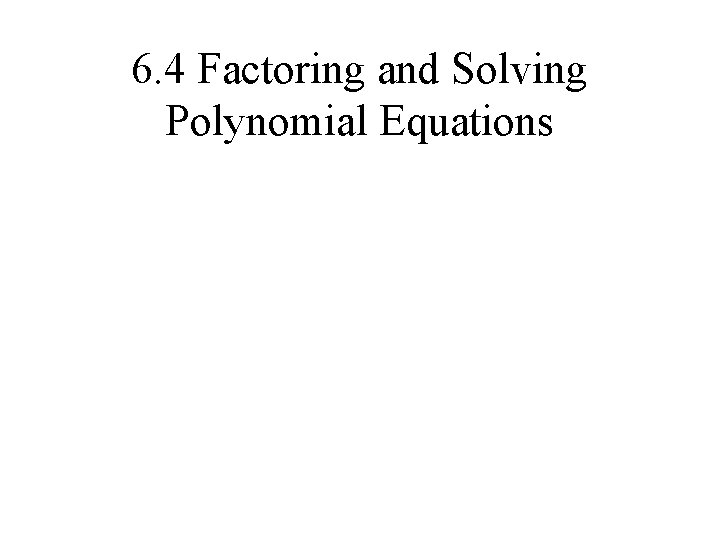6. 4 Factoring and Solving Polynomial Equations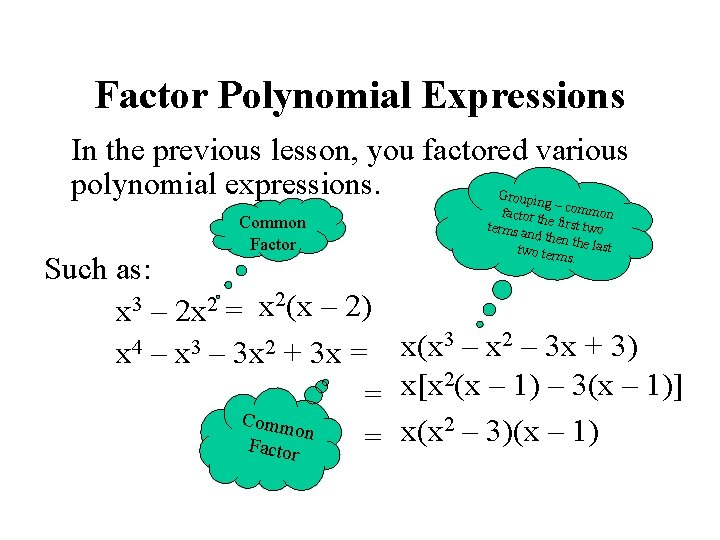Factor Polynomial Expressions In the previous lesson, you factored various polynomial expressions. Groupin g– Common Factor factor th common terms a e first two nd then t two term he last s. Such as: 2(x – 2) 3 2 x x – 2 x = 3 – x 2 – 3 x + 3) 4 3 2 x(x x – 3 x + 3 x = 2(x – 1) – 3(x – 1)] x[x = Comm 2 – 3)(x – 1) on x(x = Factor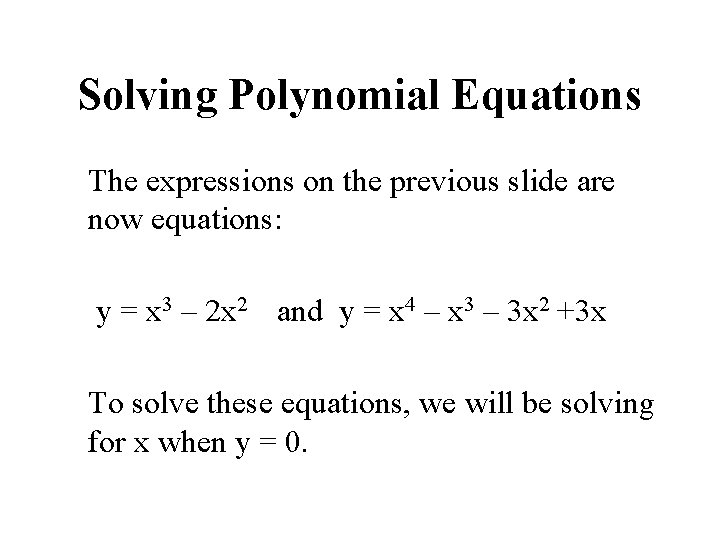Solving Polynomial Equations The expressions on the previous slide are now equations: y = x 3 – 2 x 2 and y = x 4 – x 3 – 3 x 2 +3 x To solve these equations, we will be solving for x when y = 0.Solve y = x 3 – 2 x 2 0 = x 2(x – 2) Let y = 0 Common factor Separate the factors and set them equal to zero. x 2 = 0 or x – 2 = 0 x=2 Solve for x Therefore, the roots are 0 and 2.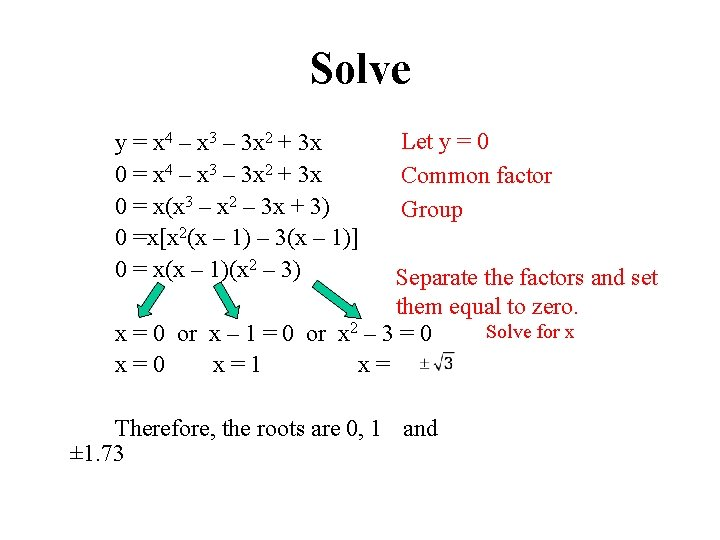Solve y = x 4 – x 3 – 3 x 2 + 3 x 0 = x(x 3 – x 2 – 3 x + 3) 0 =x[x 2(x – 1) – 3(x – 1)] 0 = x(x – 1)(x 2 – 3) Let y = 0 Common factor Group Separate the factors and set them equal to zero. Solve for x x = 0 or x – 1 = 0 or x 2 – 3 = 0 x=1 x= Therefore, the roots are 0, 1 and ± 1. 73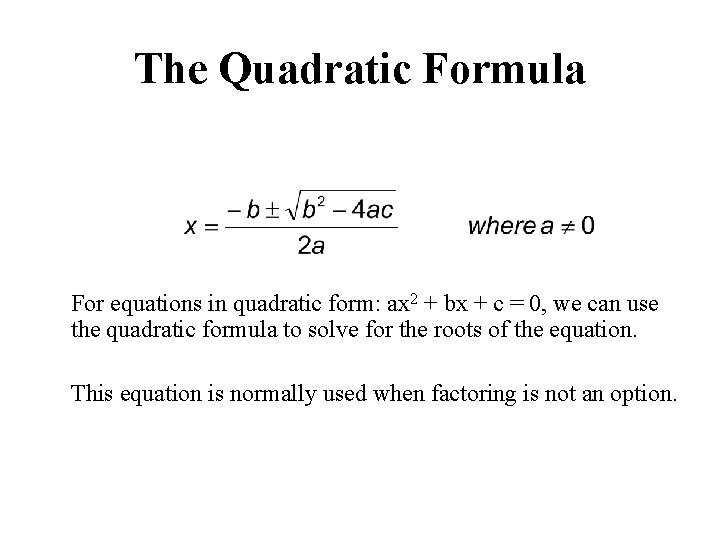The Quadratic Formula For equations in quadratic form: ax 2 + bx + c = 0, we can use the quadratic formula to solve for the roots of the equation. This equation is normally used when factoring is not an option.Using the Quadratic Formula Solve the following cubic equation: Can this equation be to solve for x We still need y = x 3 + 5 x 2 – 9 x factored? here. Can this equation be 0 =YES x(xit 2 can + 5 x– – 9) factored? factor. x =common 0 x 2 + 5 x – 9 = 0 No. however, There are no twothe quadratic formula. We can, use integers that will multiply a = 1 to -9 and add to 5. b=5 c = -9 Remember, the root 0 came from an earlier step. Therefore, the roots are 0, 6. 41 and 1. 41.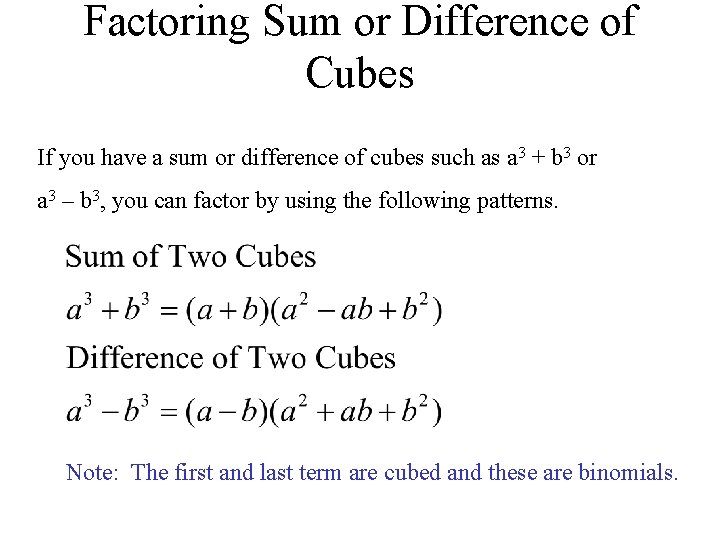Factoring Sum or Difference of Cubes If you have a sum or difference of cubes such as a 3 + b 3 or a 3 – b 3, you can factor by using the following patterns. Note: The first and last term are cubed and these are binomials.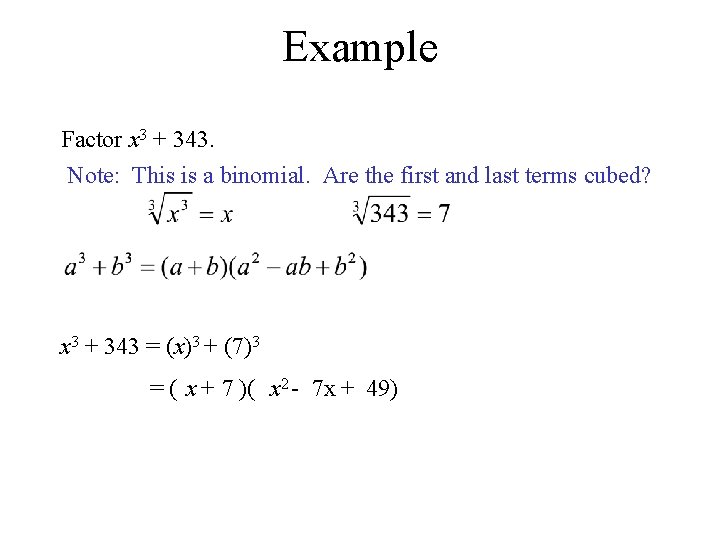Example Factor x 3 + 343. Note: This is a binomial. Are the first and last terms cubed? x 3 + 343 = (x)3 + (7)3 = ( x + 7 )( x 2 - 7 x + 49)Example Factor 64 a 4 – 27 a = a(64 a 3 – 27) Note: Binomial. Is the first and last terms cubes? = a( (4 a)3 – (3)3) Note: = a( 4 a - 3)( 16 a 2 + 12 a + 9 )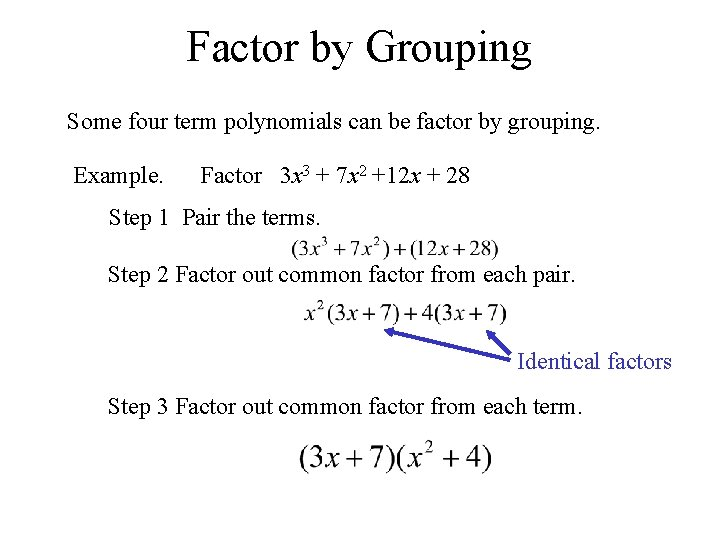Factor by Grouping Some four term polynomials can be factor by grouping. Example. Factor 3 x 3 + 7 x 2 +12 x + 28 Step 1 Pair the terms. Step 2 Factor out common factor from each pair. Identical factors Step 3 Factor out common factor from each term.Example Factor 3 x 3 + 7 x 2 -12 x - 28 Step 1 Note: Subtraction is the same as adding a negative Step 2 Step 3 Note: This factor can be further factored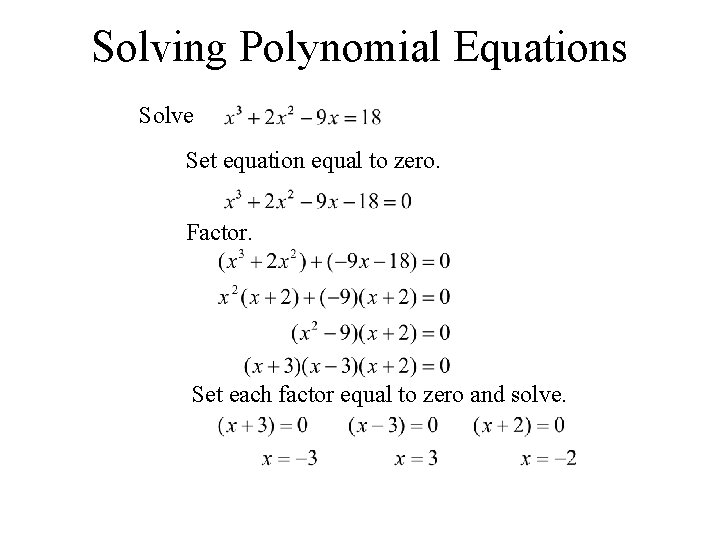Solving Polynomial Equations Solve Set equation equal to zero. Factor. Set each factor equal to zero and solve.